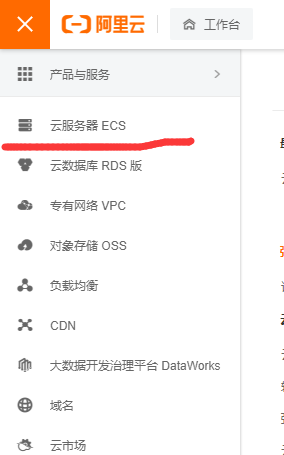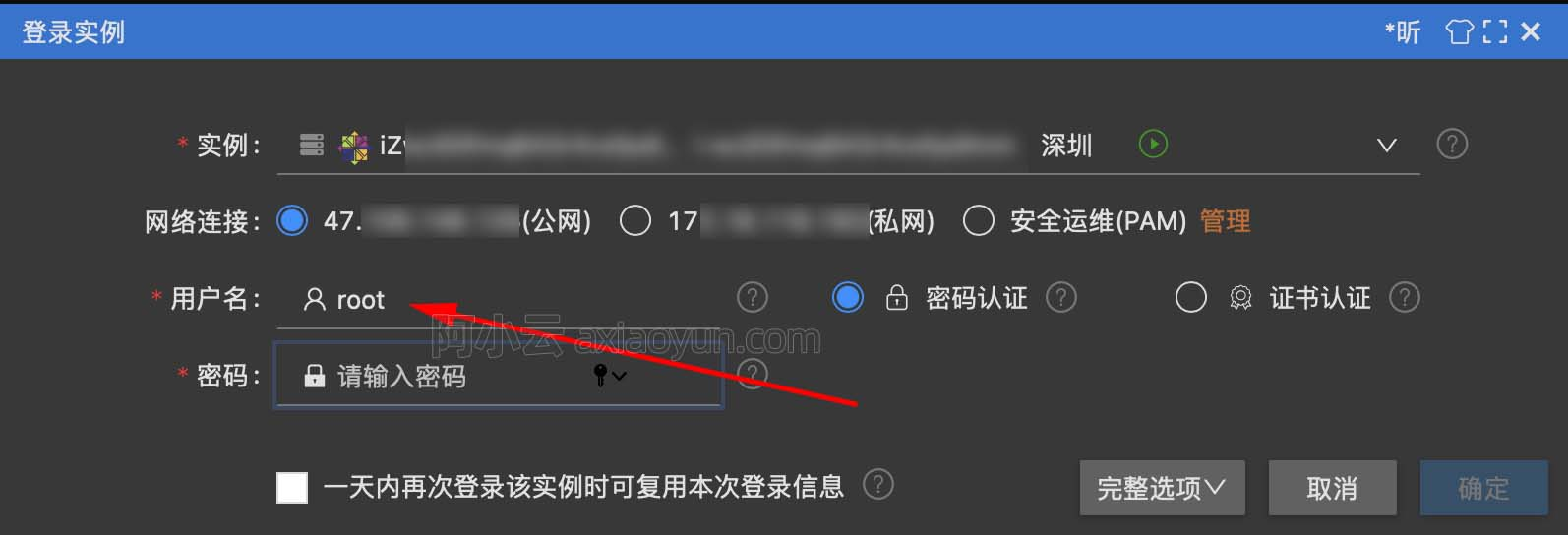成功解决To fix this you could try to: 1. loosen the range of package versions you‘ve specified ???????

+关注继续查看

解决问题he conflict is caused by:

tensorflow 2.5.0 depends on keras—nightly=2.5.0.dev

tensorflow 2.4.2 depends on tensorflow—estimator<2.5.0 and >=2.4.0

tensorflow 2.4.1 depends on tensorflow—estimator<2.5.0 and >=2.4.0

tensorflow 2.4.0 depends on tensorflow—estimator<2.5.0 and >=2.4.0rc0

tensorflow 2.3.3 depends on tensorflow—estimator<2.4.0 and >=2.3.0

tensorflow 2.3.2 depends on tensorflow—estimator<2.4.0 and >=2.3.0

tensorflow 2.3.1 depends on tensorflow—estimator<2.4.0 and >=2.3.0

tensorflow 2.3.0 depends on tensorflow—estimator<2.4.0 and >=2.3.0

tensorflow 2.2.3 depends on tensorflow—estimator<2.3.0 and >=2.2.0

tensorflow 2.2.2 depends on tensorflow—estimator<2.3.0 and >=2.2.0

tensorflow 2.2.1 depends on tensorflow—estimator<2.3.0 and >=2.2.0

tensorflow 2.2.0 depends on tensorflow—estimator<2.3.0 and >=2.2.0

To fix this you could try to:

1. loosen the range of package versions you've specified

2. remove package versions to allow pip attempt to solve the dependency conflict

解决思路

1. 放宽您指定的包版本的范围

2. 删除包版本以允许PIP尝试解决依赖项冲突

解决方法9539 06375 014389 02465 013238 0windows server 2008阿里云ECS服务器安全设置

9066 06947 04053 021954 054337 0

1701

0

《2021云上架构与运维峰会演讲合集》

《零基础CSS入门教程》

《零基础HTML入门教程》